# Si-Waveguide Absorption-Based Methane Sensor

## Tool Used: OptSim Circuit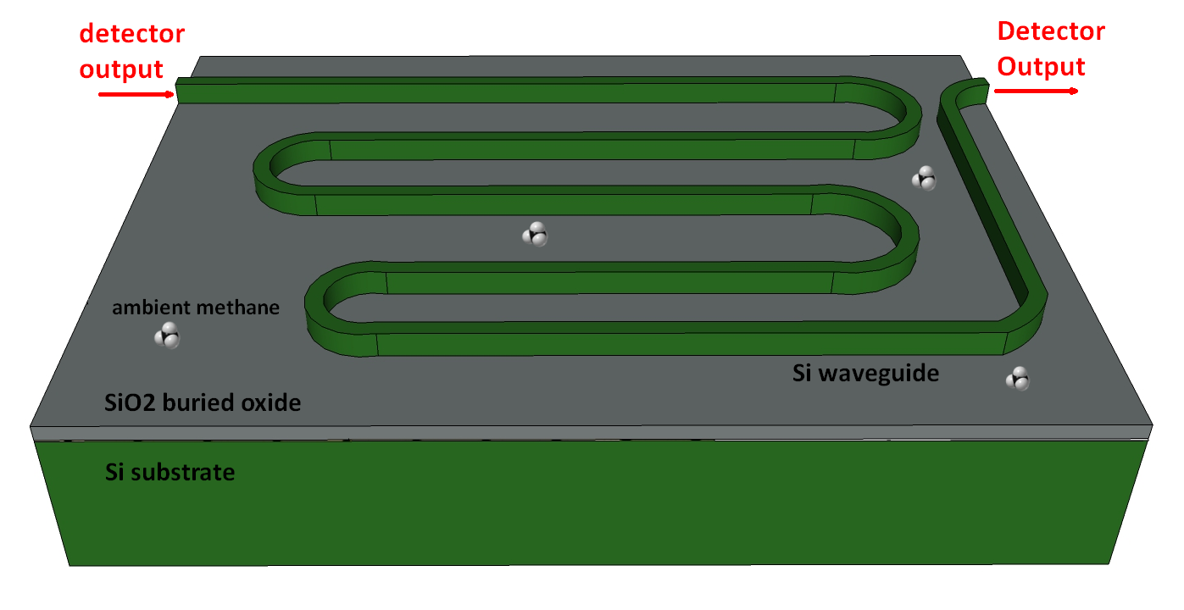In this OptSim Circuit application note, we will demonstrate an Absorption-based methane sensor using Si waveguide. This methane sensor will:

• Probe the 1650.96-nm line in methane absorption spectrum (𝛼_𝑔𝑎𝑠= 0.4347 cm-1)
• Have the following waveguide parameters:
• length 𝐿 = 10 cm,
• intrinsic loss 𝛼_𝑤𝑔 = 2 dB/cm
• methane mode overlap Γ = 25.5%
• Demonstrate 8-dB input/output coupling
• Demonstrate a measured detection limit of 100 ppmv for 60-second averaging time

## OptSim Circuit Cppmv Measurement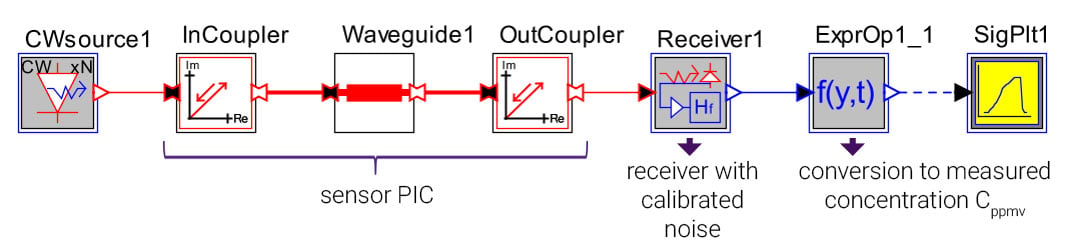• Launched power is 10 dBm at 1650.9554 nm
• Total waveguide power transmission: 𝑒−𝛼𝑤𝑔𝐿∙𝑒−Γ𝛼𝑔𝑎𝑠𝐶𝐿, where 𝐶=𝐶𝑝𝑝𝑚𝑣/106
• Receiver noise, assumed to be independent of input signal level, calibrated for 100-ppmv detection limit with 60-second averaging time
• Receiver calibration based on calculated time-averaged noise-equivalent power (NEP) and absorbance (𝑁𝐸𝐴):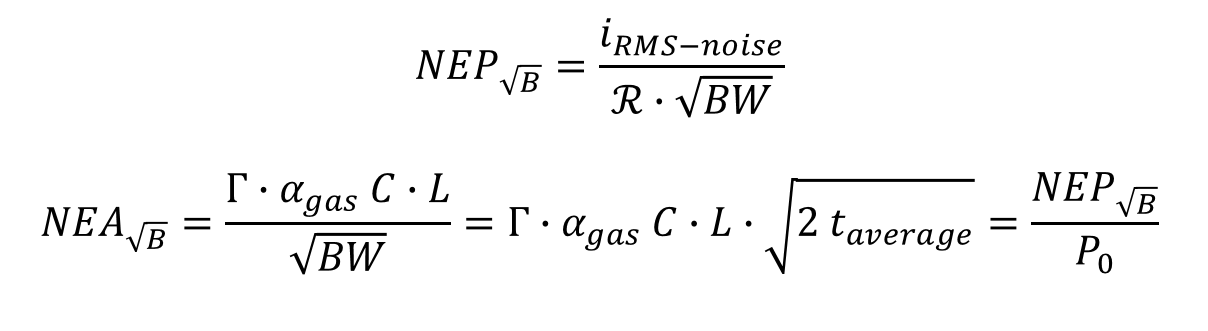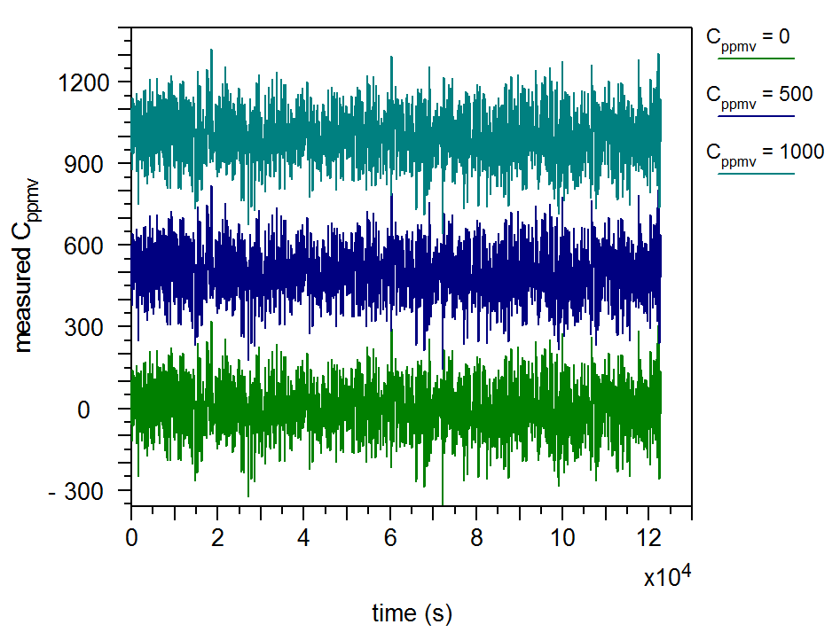## OptSim Circuit Allan Deviation Analysis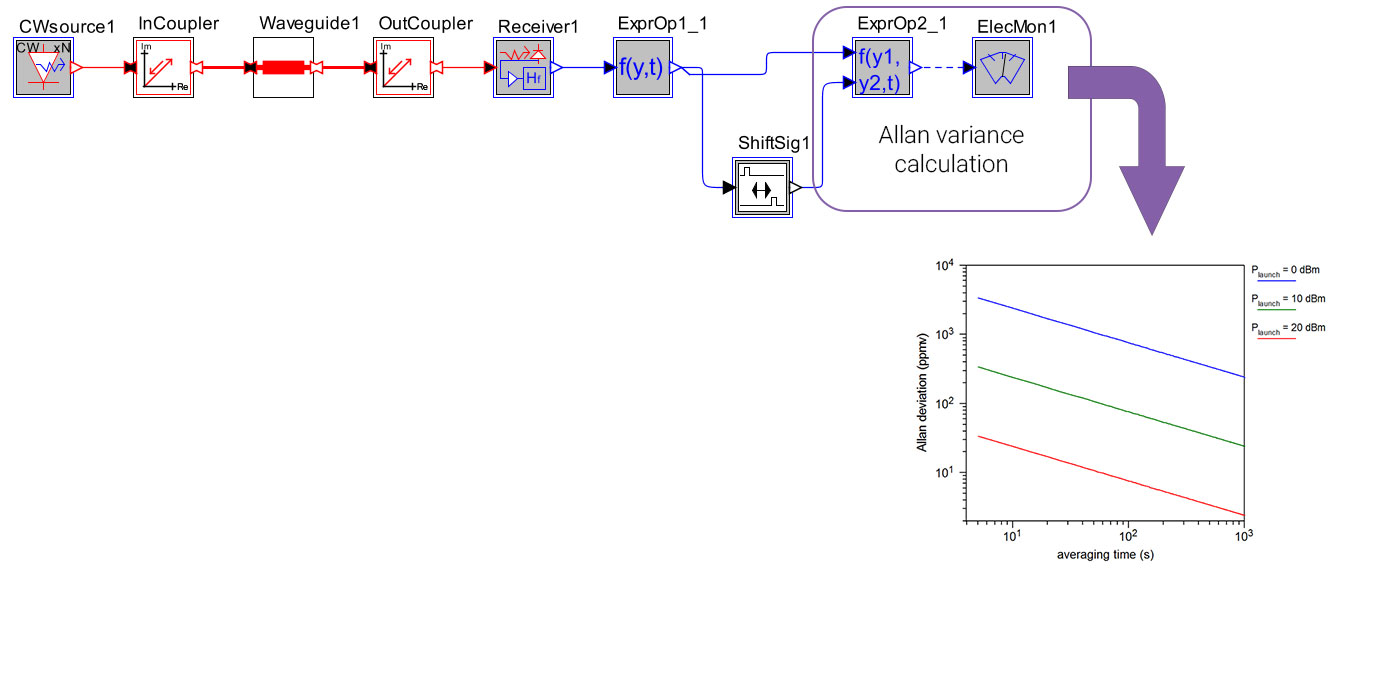Indicated blocks produce electrical signal whose average power is the Allan variance in ppmv²: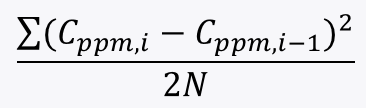where 𝐶𝑝𝑝𝑚,𝑖 is the 𝑖th measurement sample (𝑁 total) with sampling time 𝑡𝑎𝑣𝑒𝑟𝑎𝑔𝑒
• Allan deviation (ppmv) versus averaging time matches results from Tombez et al. for 10-dBm launch power
• Detection limit improves (worsens) for higher (lower) launch power – consistent with assumption that sensor noise is independent of signal power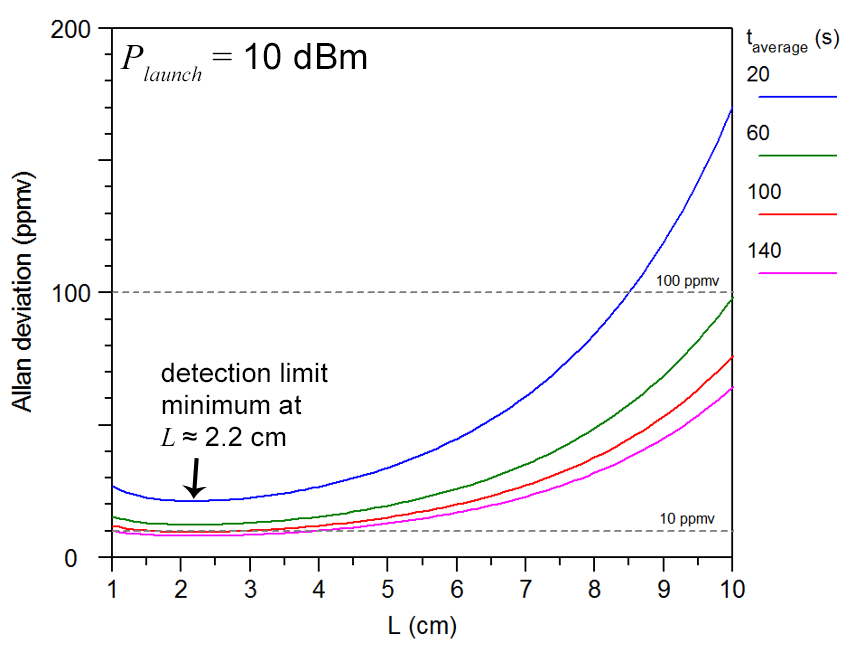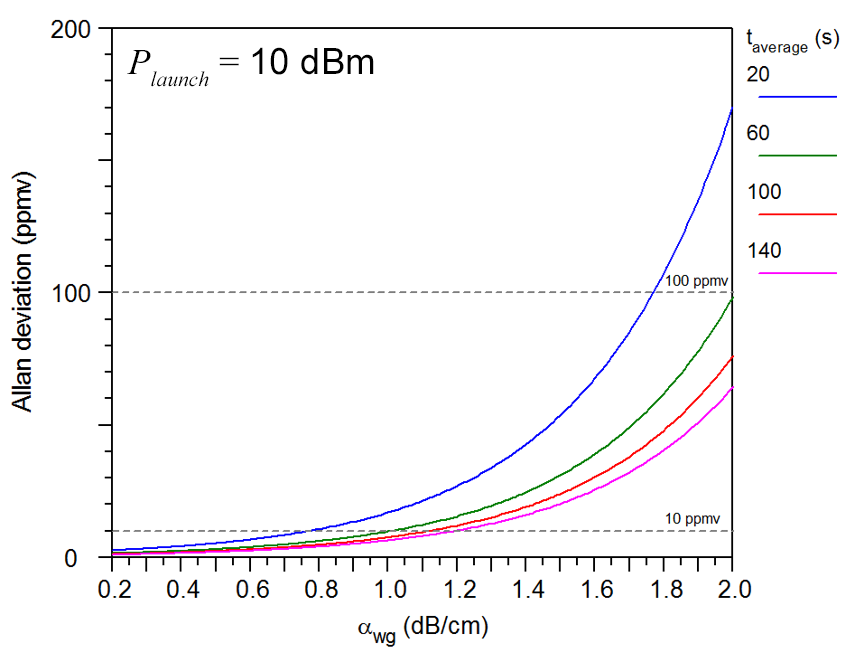• Detection limit optimum at waveguide length = 1/𝛼𝑤𝑔 [see H. Lin et al., “Mid-infrared integrated photonics on silicon: a perspective”, Nanophotonics 7(2), 393-420 (2018).]
• Sub-10-ppmv detection limit at optimum length for averaging times ≳ 91 seconds
• Detection limit improves as waveguide loss decreases
• Results assume sensor noise independent of signal power levels